# Texas Go Math Grade 5 Lesson 3.4 Answer Key Multiply Using Expanded Form

Refer to our Texas Go Math Grade 5 Answer Key Pdf to score good marks in the exams.
Test yourself by practicing the problems from Texas Go Math Grade 5 Lesson 3.4 Answer Key
Multiply Using Expanded Form.

## Texas Go Math Grade 5 Lesson 3.4 Answer Key Multiply Using Expanded Form

Essential Question
How can you use expanded form and place, value to multiply a decimal and a whole number?

Unlock the Problem

The length of a day is the amount of time it takes a planet to make a complete a rotation on its axis.
On Jupiter, there are 9.8 Earth hours in a day. How many Earth hours are there in 46 days on Jupiter?

You can use a model and partial products to solve the problem.

One Way
Use a model.
Multiply. 46 × 9.8
There are _450.8__ Earth hours In 46 days on Jupiter,

Explanation: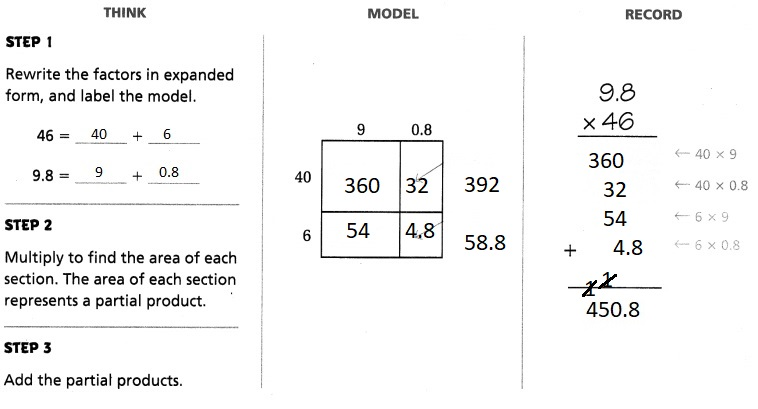Question 1.
What if you wanted to find the number of Earth hours in 125 days on Jupiter?
There are 1225 Earth hours In 125 days on Jupiter,

Explanation: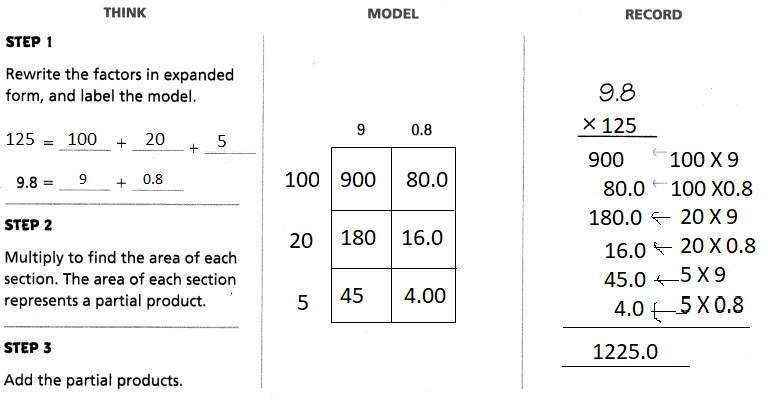On Jupiter there are 9.8 Earth hours in a day,
So Earth hours are there in 125 days on Jupiter are 1225.0.

Another Way
Use place value patterns.
A day on the planet Mercury lasts about 58.6 Earth days.
How many Earth days are there in 14 days on Mercury?

Multiply. 14 × 58.6

Step 1
Write the decimal factor as a whole number.

Step 2
Multiply as with whole numbers.

Step 3
Place the decimal point.
The decimal product is _812__ of the whole number product.
So, there are _820.40__ Earth days in 14 days on Mercury.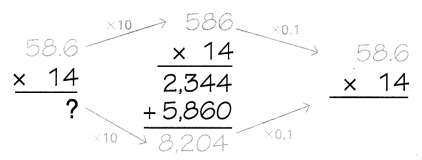There are 820.4 Earth days in 14 days on Mercury,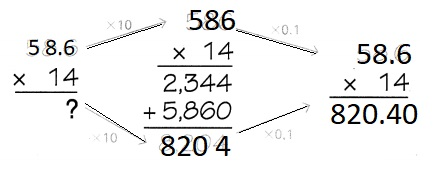Explanation:
Multiplying 14 × 58.6

Step 1
Writing the decimal factor as a whole number as 586,

Step 2
Multiplying 586 as with whole numbers 14,

Step 3
Place the decimal point.
The decimal product is __820.40_ of the whole number product.
So, there are _820.40__ Earth days in 14 days on Mercury.

Math Talk
Mathematical Processes
What if you rewrite the problem as (10 + 4) × 58.6 and
use the Distributive Property to solve?
Explain how this is similar to your model using place value.
By using distributive property there are 820.40 Earth days in 14 days on Mercury,

Explanation:
Given to multiply 14 × 58.6 by using distributive property,
=(10 + 4) X 58.6 = (10 X 58.6) + (4 X 58.6),
= 586.0 + 234.40,
= 820.40.
Share and Show

Draw a model to find the product.

Question 1.
19 × 0.75 = _14.25___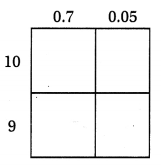Explanation:
Given 19 × 0.75 =

Step 1
Writing the decimal factor as a whole number as 75,

Step 2
Multiplying 75 as with whole numbers 19,

Step 3
Place the decimal point.
The decimal product is 14.25 of the whole number product.

Question 2.
27 × 8.3 = 224.1
27 X 8.3 = 224.1,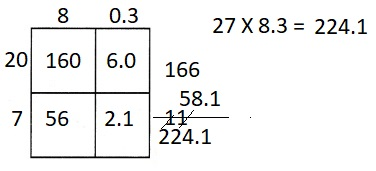Explanation:
Given 27 × 8.3 =

Step 1
Writing the decimal factor as a whole number as 83,

Step 2
Multiplying 83 as with whole numbers 27,

Step 3
Place the decimal point.
The decimal product is 224.1 of the whole number product.

Find the product.

Question 3.
18 × 8.7 = _156.6__
18 X 8.7 = 156.6,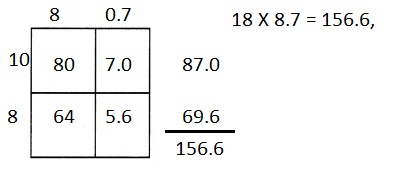Explanation:
Given 18 × 8.7 =

Step 1
Writing the decimal factor as a whole number as 87,

Step 2
Multiplying 87 as with whole numbers 18,

Step 3
Place the decimal point.
The decimal product is 156.6 of the whole number product.

Question 4.
23 × 56.1 =
23 X 56.1 = 1,290.3,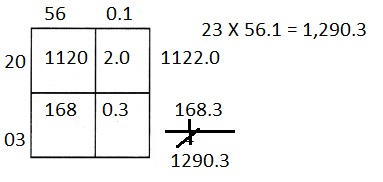Explanation:
Given 23 × 56.1 =

Step 1
Writing the decimal factor as a whole number as 561,

Step 2
Multiplying 561 as with whole numbers 23,

Step 3
Place the decimal point.
The decimal product is 1,290.3 of the whole number product.

Question 5.
47 × 5.92 = _278.24__
47 X 5.92 = 278.24,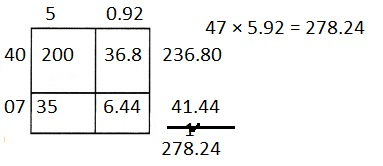Explanation:
Given 47 × 5.92 =

Step 1
Writing the decimal factor as a whole number as 592,

Step 2
Multiplying 592 as with whole numbers 47,

Step 3
Place the decimal point.
The decimal product is 278.24 of the whole number product.

Unlock the Problem

Question 6.
H.O.T. While researching facts on the planet Earth,
Kate learned that a true Earth day is about 23.93 hours long.
How many hours are in 2 weeks on Earth?

a. What are you being asked to find?
Number of hours are in 2 weeks on Earth,

Explanation:
Given to find how many hours are in 2 weeks on Earth?.

b. What information do you need to know to solve the problem?
True Earth day is about 23.93 hours long,

Explanation:
Given Kate learned that a true Earth day is about 23.93 hours long.

c. Write an expression to represent the problem to be solved.
Expression is 2 X 7 X 23.93 hours,

Explanation:
As given true Earth day is about 23.93 hours long and number of
hours in 2 weeks( 2 weeks X 7 days) on Earth.

d. Show the steps you used to solve the problem.
2 X 7 X 23.93 = 335.02,

Explanation:
131
23.93
X   14
095.72
239.30
335.02

e. Complete the sentences.
On Earth, there are about __23.93_ hours in a day __7_ days in 1 week and
_14__ days in two weeks.
Since _14__ × _23.93_ = _335.02__ , there are about _335.02__ hours in 2 weeks on Earth.

There are about 335.02 hours in 2 weeks on Earth,

Explanation:
Given on Earth, there are about 23.9 hours in a day 7 days in 1 week and
14 days in two weeks.
Since 14 × 23.93 = 335.02 , there are about 335.02 hours in 2 weeks on Earth.

Question 7.
H.O.T. Multi-Step A jacket costs $40 at the store. Max pays only 0.7 of the price because his father works at the store. Evan has a coupon for$10 off.
Explain Who will pay the least for the jacket?Max will pay $28 the least for the jacket, Explanation: Given a jacket costs$40 at the store. Max pays only 0.7 of the price
because his father works at the store. So Max pays 0.7 X $40 =$28,
Evan has a coupon for $10 off, Evan has$40 – $10 =$30, Upon
compairing Max and Evan- $28 and$30, as $28 >$30,
Max will pay the least for the jacket.

Question 8.
In the rain forest, you find a beetle. You use beads to measure the beetle.
It only measures 11 beads long. If the length of each bead is 12.8 mm,
what is the length of this beetle?
(A) 128 mm
(B) 140.8 mm
(C) 157.7 mm
(D) 166.4 mm
(B) 140.8 mm,

Explanation:
Given in the rain forest we find a beetle.
We use beads to measure the beetle.
It only measures 11 beads long and if the length of each bead is 12.8 mm,
The length of this beetle is 11 X 12.8 = 140.8 mm which matches with (B).

Question 9.
Use Math Language Which would help you find the solution to 12 × 4.7?
(A) Multiply 10 and 2 each by 4 and 0.7. Then add.
(B) Multiply 4.7 by 1, and 4.7 by 2. Then add.
(C) Multiply 1 by 4, and 2 by 0.7. Then add.
(D) Multiply 1 and 0.2 each by 40 and 0.7. Then add.
(A) Multiply 10 and 2 each by 4 and 0.7. Then add,

Explanation:
Given to find the solution to 12 X 4.7
1
12
X 4.7
Multiply 10 and 2 each by 4 and 0.7 then add which matches (A).

Question 10.
Multi-Step Alonzo wanted to find the solution to 23 × 12.53.
He made the area model shown below. Where did he go wrong in his calculations?
240 + 36 + 106 + 15.9 = 397.9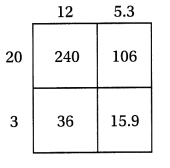(A) Alonzo is correct.
(B) He used 20 and 3 in the area model.
(C) He found the sum of the values in the area model incorrectly.
(D) He used 5.3 in the area model instead of 0.53.
(A) Alonzo is correct,

Explanation:
Given Alonzo wanted to find the solution to 23 × 12.53.
He made the area model shown above, He did not do wrong in his calculations
240 + 36 + 106 + 15.9 = 397.9, therefore Alonzo is correct.

Texas Test Prep

Question 11.
A car travels 56.7 miles in an hour. If it continues at the same speed,
how far will the car travel in 12 hours?
(A) 6,804 miles
(B) 680.04 miles
(C) 680.4 miles
(D) 68.004 miles
(C) 680.4 miles,

Explanation:
Given a car travels 56.7 miles in an hour If it continues at the same speed,
the car will travel in 12 hours as 12 X56.7 =
56.7
X 12
113.4
567.0
680.4,
matches with (C).

### Texas Go Math Grade 5 Lesson 3.4 Homework and Practice Answer Key

Draw a model to find the product.

Question 1.
21 × 0.64 = ___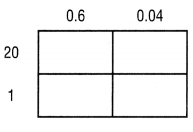21 × 0.64 = 13.44,

Explanation:
Given 21 X 0.64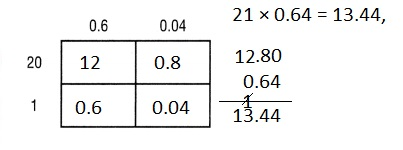we get 21 × 0.64 = 13.44.

Question 2.
16 × 3.7 = ___16 X 3.7 = 59.2,

Explanation:
Given 16 X 3.7 =we get 16 X 3.7 = 59.2.

Find the product.

Question 3.
42 × 7.2 = 302.4___
42 × 7.2 = 302.4,

Explanation:
Given 42 × 7.2 =we get 302.4.

Question 4.
28 × 3.17 = 88.76 ____
28 × 3.17 = 88.76,

Explanation:
Given 28 × 3.17 =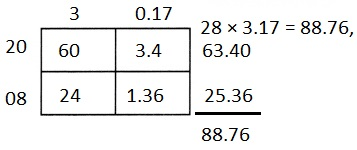we get 88.76.

Question 5.
34 × 0.41 = 13.94 ___
34 × 0.41 = 13.94,

Explanation:
Given 34 X 0.41 =we get 34 X 0.41 = 13.94.

Problem Solving

Question 6.
Craig works at a restaurant for 21 hours in January.
At this rate, how many hours will he work after 7.5 months?
Approximately Craig works for 157.5 hours,

Explanation:
Given Craig works at a restaurant for 21 hours in January.
At this rate, Number of hours will he work after 7.5 months is
21 hours X 7.5 =
7.5
X21
007.5
150.0
157.5,
Therefore, Craig works approximately for 157.5 hours.

Question 7.
Lori’s dog eats 3.82 pounds of food in one week.
How much food will the dog eat in 18 weeks?
The dog will eat 68.76 pounds in 18 weeks,

Explanation:
Given Lori’s dog eats 3.82 pounds of food in one week,
Food will the dog eat in 18 weeks is
3.82 X 18 =
18
X3.82
00.36
14.40
54.00
68.76, So the dog will eat 68.7 pounds.

Lesson Check

Question 8.
Amid needs 32.9 meters of fabric to make one tent.
He has an order for 12 tents.
How many meters of fabric does he need?
(A) 367.2 meters
(B) 394.8 meters
(C) 288.3 meters
(D) 416.9 meters
(B) 394.8 meters,

Explanation:
Given Amid needs 32.9 meters of fabric to make one tent.
He has an order for 12 tents, meters of fabric does he need is
32.9 X 12 =
12
X32.9
065.8
329.0
394.8 ,
which matches with (B).

Question 9.
Angeline fills one water jug with 5.8 gallons of water.
She needs to fill 17 water jugs for day camp.
How many gallons of water does she need?
(A) 114.2 gallons
(B) 82.5 gallons
(C) 98.6 gallons
(D) 107.9 gallons
(C) 98.6 gallons,

Explanation:
Given Angeline fills one water jug with 5.8 gallons of water.
She needs to fill 17 water jugs for day camp.
Therefore gallons of water does she need is
5.8 X 17 =
17
X5.8
13.6
85.0
98.6,
which matches with (C).

Question 10.
Corey rides his bike 17.4 miles in one hour. If he rides this distance every day,
how far will he ride in 11 days?
(A) 191.4 miles
(B) 187.1 miles
(C) 213.8 miles
(D) 176.4 miles
(A) 191.4 miles,

Explanation:
Given rides  his bike 17.4 miles in one hour,
if he rides this distance every day far will he ride in 11 days,
17.4 miles X 11 =
11
X17.4
017.40
174.00
191.4
, matches with (A).

Question 11.
Kavita’s younger sister measures objects by using paper clips that are 16.2 mm long.
Kavita’s desk is 76 paper clips wide. What is the width of Kavita’s desk?
(A) 1,394.9 mm
(B) 1,231.2 mm
(C) 1,230.8 mm
(D) 1,213.2 mm
(B) 1,231.2 mm,

Explanation:
Given Kavita’s younger sister measures objects by using
paper clips that are 16.2 mm long.
Kavita’s desk is 76 paper clips wide.
So the width of Kavita’s desk is 16.2 X 76 =
76
X16.2
015.2
456.0
760.0
1231.2,
matches with (B).

Question 12.
Multi-Step Max makes an expanded form model to find the solution to 23 × 11.09.
Which calculation reflects the numbers in his model?
(A) 220 + 34 + 18 + .27
(B) 220 + 22 + 1.8 + 2.7
(C) 217 + 33 + 1.8 + .27
(D) 220 + 33 + 1.8 + .27
(D) 220 + 33 + 1.8 + .27 calculation reflects the given model,

Explanation:
Given Max makes an expanded form model to find the solution to 23 × 11.09 =
11.09
X 23
033.27
221.80
255.07
Now checking with bits
(A) 220 + 34 + 18 + .27 = 272.27,
(B) 220 + 22 + 1.8 + 2.7 = 246.5,
(C) 217 + 33 + 1.8 + .27 = 252.07,
(D) 220 + 33 + 1.8 + .27 = 255.07
So bit (D) calculation reflects the given model.

Question 13.
Multi-Step Kim makes an expanded form model to find the solution to 18 × 14.39.
The calculation shows the numbers in each box of the model.
Which number in the calculation is incorrect?
140 + 112 + 3.09 + 3.12
(A) 140
(B) 112
(C) 3.09
(D) 3.12
(D) 3.12,

Explanation:
Given Kim makes an expanded form model to find the solution to 18 × 14.39,
14.39
X 18
115.12
143.90
259.02

The calculation shows the numbers in each box of the model
140 + 112 + 3.09 + 3.12 = 258.21,
The difference is 259.02 – 258.21 = 0.81,
The number in the calculation is incorrect
if we consider (A) we have to take maximum round value 1 which is more than 0.81,
result is (140 + 1) + 112 + 3.09 + 3.12 = 259.21 ≠ 259.02,
if we consider (B) we have to take maximum round value 1 which is more than 0.81,
result is 140 + (112 + 1) + 3.09 + 3.12 = 259.21 ≠ 259.02,
if we consider (C) we have to take maximum round value 0.01 which is lessthan 0.81,
140 + 112 + (3.09 + 0.01) + 3.12 = 258.22 ≠ 259.02,
if we consider (D) we have to take maximum round value 1 which is more 0.81,
140 + 112 + 3.09 + (3.12 + 0.81) = 259.02 = 259.02,
matches with (D).

Scroll to Top